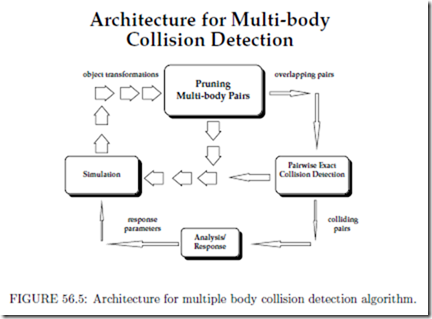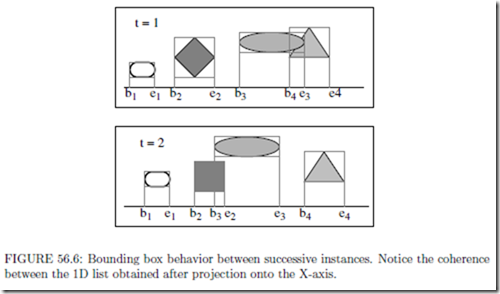# Collision Detection:Large Environments

###### Large Environments

Large environments are composed of multiple moving objects. Diﬀerent methods have been proposed to overcome the computational bottleneck of O(n2) pairwise tests in an environment composed of n objects. The problem of performing proximity queries in large environments, is typically divided into two parts [3, 23]: the broad phase, in which we identify the pair of objects on which we need to perform diﬀerent proximity queries, and the narrow phase, in which we perform the exact pairwise queries. In this section, we present a brief overview of algorithms used in the broad phase.

The simplest algorithms for large environments are based on spatial subdivisions. The space is divided into cells of equal volume, and at each instance the objects are assigned to one or more cells. Collisions are checked between all object pairs belonging to eachcell. In fact, Overmars has presented an eﬃcient algorithm based on hash table to eﬃcient perform point location queries in fat subdivisions . This approach works well for sparse environments in which the objects are uniformly distributed through the space. Another approach operates directly on four-dimensional volumes swept out by object motion over time .

Multiple-Object Collision Detection

Large-scale environments consist of stationary as well as moving objects. Let there be N moving objects and M stationary objects. Each of the N moving objects can collide with the other moving objects, as well as with the stationary ones. Keeping track of ( N 2 + NM pairs of objects at every time step can become time consuming as N and M get large. To achieve interactive rates, we must reduce this number before performing pairwise collision tests. In this section, we give an overview of sweep-and-prune algorithm used to perform multiple-object collision detection . The overall architecture of the multiple object collision detection algorithm is shown in Fig. 56.5.1.

The algorithm uses sorting to prune the number of pairs. Each object is surrounded by a 3-dimensional bounding volume. These bounding volumes are sorted in 3-space to determine which pairs are overlapping. The algorithm only needs to perform exact pairwise collision tests on these remaining pairs.

However, it is not intuitively obvious how to sort objects in 3-space. The algorithm uses a dimension reduction approach . If two bodies collide in a 3-dimensional space, their orthogonal projections onto the xy, yz, and xz-planes and x, y, and z-axes must overlap. Based on this observation, the algorithm uses axis-aligned bounding boxes and eﬃciently project them onto a lower dimension, and perform sorting on these lower-dimensional structures.

The algorithm computes a rectangular bounding box to be the tightest axis-aligned box containing each object at a particular orientation. It is deﬁned by its minimum and max- imum x, y, and z-coordinates. As an object moves, the algorithm recomputes its minima and maxima, taking into account the object’s orientation.

As a precomputation, the algorithm computes each object’s initial minima and maxima along each axis. It is assumed that the objects are convex. For non-convex polyhedral models, the following algorithm is applied to their convex hulls. As an object moves, its minima and maxima are recomputed in the following manner:

1. Check to see if the current minimum (or maximum) vertex for the x, y, or z- coordinate still has the smallest (or largest) value in comparison to its neighboring vertices. If so the algorithm terminates.

2. Update the vertex for that extreme direction by replacing it with the neighboring vertex with the smallest (or largest) value of all neighboring vertices. Repeat the entire process as necessary.

This algorithm recomputes the bounding boxes at an expected constant rate. It exploits the temporal and geometric coherence.

The algorithm does not transform all the vertices as the objects undergo motion. As it is updating the bounding boxes, new positions are computed for current vertices using matrix- vector multiplications. This approach is optimized based on the fact that the algorithm is interested in one coordinate value of each extremal vertex, say the x coordinate while updating the minimum or maximum value along the x-axis. Therefore, there is no need to transform the other coordinates in order to compare neighboring vertices. This reduces the number of arithmetic operations by two-thirds, as we only compute a dot-product of two vectors and do not perform matrix-vector multiplication.

OneDimensional Sweep and Prune

The one-dimensional sweep and prune algorithm begins by projecting each three-dimensional bounding box onto the x, y, and z axes. Because the bounding boxes are axis-aligned, projecting them onto the coordinate axes results in intervals (see Fig. 56.5.1).

The goal is to compute the overlaps among these intervals, because a pair of bounding boxes can overlap if and only if their intervals overlap in all three dimensions.

The algorithm constructs three lists, one for each dimension. Each list contains the values of the endpoints of the intervals corresponding to that dimension. By sorting these lists, it determines which intervals overlap. In the general case, such a sort would take O(n log n) time, where n is the number of objects. This time bound is reduced by keeping the sorted lists from the previous frame, changing only the values of the interval endpoints.

In environments where the objects make relatively small movements between frames, the lists will be nearly sorted, so we can sort in expected O(n) time, as shown in [50, 51]. In practice, insertion sort works well for almost sorted lists .

In addition to sorting, the algorithm keeps track of changes in the overlap status of interval pairs (i.e. from overlapping in the last time step to non-overlapping in the current ti mestep, and vice-versa). This can be done in O(n + ex + ey + ez ) time, where ex, ey , and ez are the number of exchanges along the x, y, and z-axes. This also runs in expected linear time due to coherence, but in the worst case ex, ey , and ez can each be O(n2) with an extremely small constant.

This algorithm is suitable for dynamic environments where coherence between successive static queries is preserved. In computational geometry literature several algorithms exist that solve the static version of determining 3-D bounding box overlaps in O(n log2 n + s)time, where s is the number of pairwise overlaps [52, 53]. It has been reduced to to O(n + s) by exploiting coherence.

Implementation and Application

The sweep-and-prune has been used in some of the widely uses collision detection systems, including I-COLLIDE, V-COLLIDE, SWIFT and SWIFT++ . It has been used for multi-body simulations and interactive walkthroughs of complex environments.

Two-Dimensional Intersection Tests

The two-dimensional intersection algorithm begins by projecting each three-dimensional axis-aligned bounding box onto any two of the x-y, x-z, and y-z planes. Each of these projections is a rectangle in 2-space. Typically there are fewer overlaps of these 2-D rectangles than of the 1-D intervals used by the sweep and prune technique. This results in fewer swaps as the objects move. In situations where the projections onto one-dimension result in densely clustered intervals, the two-dimensional technique is more eﬃcient. The interval tree is a common data structure for performing such two-dimensional range queries .

Each query of an interval intersection takes O(log n + k) time where k is the number of reported intersections and n is the number of intervals. Therefore, reporting intersections among n rectangles can be done in O(n log n+K) where K is the total number of intersecting rectangles .